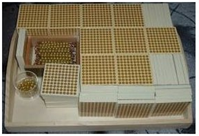ACTIVITY:

## Montessori Multiplication with the Golden Beads

MATERIALS:A set of large number cards

Three sets of small number cards

A felt lined tray for each child

2 tables – one for the beads (bank)

one for the cards

One floor mat

OBJECTIVES:

To give the child concrete experience of multiplication and to teach the child the term.

To give the child an understanding that multiplication is a form of repeated addition.

CONTROL OF ERROR:

NA

AGE: 3 – 6 years approx.
• The child collects and places the corresponding large number cards below the beads.
• The second child brings down the tens, counts them and places the corresponding number cards below them.
• The hundreds are brought down and counted and labelled as before.
• When all the beads are counted and all the corresponding quantities in number cards have been placed below them, the cards are then overlapped.
• The large overlapped cards are then placed below the small number cards, which have remained on the right hand side of the mat.
• The Montessori Directress then explains to the children that this time we added up three numbers that were exactly the same quantity. When we add three numbers that are the same, then we are multiplying the number three times. This is called multiplication.
• Re-state the operation saying, “each child collected 233 beads and we added all three numbers (or we can say we multiplied it three times) and now we have a larger number, 699.
• End the work cycle.
• Sorry, the comment form is closed at this time.

+ +